# Motion and Time (Physics) Class 7 - NCERT Questions

Q 1.

Classify the following as motion along a straight line, circular or oscillatory motion:
(i) Motion of your hands while running.
(ii) Motion of a horse pulling a cart on a straight road.
(iii) Motion of a child in a merry-go-round.
(iv) Motion of a child on a see-saw.
(v) Motion of the hammer of an electric bell.
(vi) Motion of a train on a straight bridge.

SOLUTION:

(i) Oscillatory motion (ii) Rectilinear motion (iii) Circular motion
(iv) Oscillatory motion (v) Oscillatory motion (vi) Rectilinear motion

Q 2.

Which of the following are not correct?
(i) The basic unit of time is second.
(ii) Every object moves with a constant speed.
(iii) Distances between two cities are measured in kilometres.
(iv) The time period of a given pendulum is not constant.
(v) The speed of a train is expressed in m/h.

SOLUTION:

(ii), (iv) and (v)

Q 3.

A simple pendulum takes 32 s to complete 20 oscillations. What is the time period of the pendulum?

SOLUTION: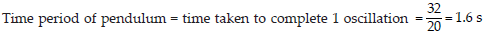Q 4.

The distance between two stations is 240 km. A train takes 4 hours to cover this distance. Calculate the speed of the train.

SOLUTION: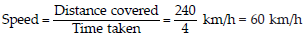Q 5.

The odometer of a car reads 57321.0 km when the clock shows the time 08:30 AM. What is the distance moved by the car, if at 08:50 AM, the odometer reading has changed to 57336.0 km? Calculate the speed of the car in km/min during this time. Express the speed in km/h also.

SOLUTION: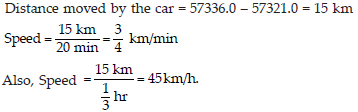Q 6.

Salma takes 15 minutes from her house to reach her school on a bicycle. If the bicycle has a speed of 2 m/s, calculate the distance between her house and the school.

SOLUTION: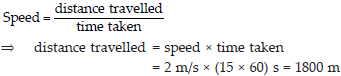Q 7.

Show the shape of the distance-time graph for the motion in the following cases:
(i) A car moving with a constant speed. (ii) A car parked on a side road.

SOLUTION: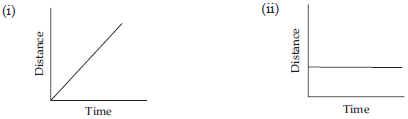Q 8.

Which of the following relations is correct?
(i) Speed = Distance × Time
(ii) Speed = Distance/Time
(iii) Speed = Time/Distance
(iv) Speed = 1/Distance × Time

SOLUTION:

(ii) Speed = Distance/Time

Q 9.

The basic unit of speed is:
(i) km/min (ii) m/min (iii) km/h (iv) m/s

SOLUTION:

As temperature of iron ball and water is same.

Q 10.

A car moves with a speed of 40 km/h for 15 minutes and then with a speed of 60 km/h for the next 15 minutes. The total distance covered by the car is:
(i) 100 km (ii) 25 km (iii) 15 km (iv) 10 km

SOLUTION: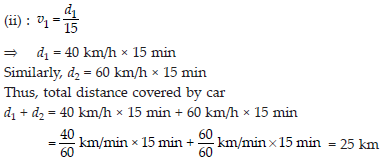Q 11.

Suppose the two photographs, shown in fig. (A) and fig. (B) had been taken at an interval of 10 seconds. If a distance of 100 metres is shown by 1 cm in these photographs, calculate the speed of the blue car.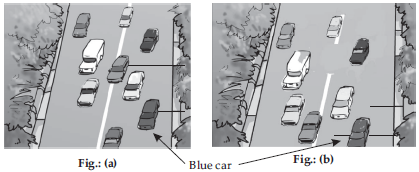SOLUTION:

14m/s

Q 12.

Figure shows the distance-time graph for the motion of two vehicles A and B. Which one of them is moving faster?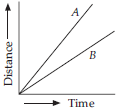SOLUTION:

As shown in distance-time graph, at any particular time, A has covered more distance than B, therefore A is moving faster.

Q 13.

Which of the following distance-time graphs shows a truck moving with speed which is not constant?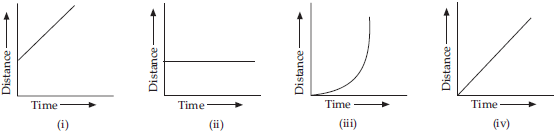SOLUTION:

(iii)